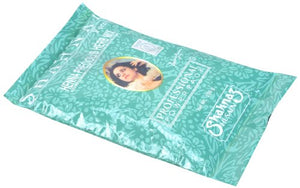# Shahnaz Husain Henna Herb Mix - 200 G

• \$22.65
• Save \$21.86

• A

• p

• o

• w

• e

• r

• f

• u

• l

• b

• l

• e

• n

• d

• o

• f

• h

• e

• n

• n

• a

• a

• n

• d

• o

• t

• h

• e

• r

• h

• e

• r

• b

• s

• f

• o

• r

• n

• a

• t

• u

• r

• a

• l

• h

• a

• i

• r

• c

• a

• r

• e

• a

• n

• d

• c

• o

• n

• d

• i

• t

• i

• o

• n

• i

• n

• g

• .

• C

• l

• e

• a

• n

• s

• e

• s

• t

• h

• e

• s

• c

• a

• l

• p

• a

• n

• d

• r

• e

• m

• o

• v

• e

• s

• l

• o

• o

• s

• e

• d

• a

• n

• d

• r

• u

• f

• f

• f

• l

• a

• k

• e

• s

• .

• E

• n

• h

• a

• n

• c

• e

• s

• t

• h

• e

• h

• e

• a

• l

• t

• h

• y

• l

• o

• o

• k

• o

• f

• t

• h

• e

• h

• a

• i

• r

• ,

• w

• i

• t

• h

• a

• t

• t

• r

• a

• c

• t

• i

• v

• e

• h

• i

• g

• h

• l

• i

• g

• h

• t

• s

• a

• n

• d

• s

• h

• e

• e

• n

• .

A powerful blend of henna and other herbs for natural hair care and conditioning. Cleanses the scalp and removes loose dandruff flakes. Enhances the healthy look of the hair, with attractive highlights and sheen.

every pages